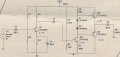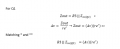# Calculate the resistance values of Class A amplifier

#### Doppler2902

Joined Sep 15, 2020
35
Hello, I have to calculate the resistance values for this Class A amplifier (R1, R2, R3, R4):So I know that voltage in the charge of 100 ohms (R9) is 6 Vrms and for calculate R1, R2, R3 and R4 I did the following:But I don't know how to operate it because I have three unknows (R3, Av and Ie for re'). Some idea for solve this? Thanks

#### Audioguru again

Joined Oct 21, 2019
4,693
With an input of 5mV peak which is 3.54mV RMS and an output of 6V RMS then you need a voltage gain of 1695 times which is impossible with the simple circuit you show,

You need a preamp with a voltage gain of about 80 times plus a power amplifier with a voltage gain of 21.2 times.
They both need much more gain so that negative feedback can reduce the severe distortion.

What is R9? Its 100 ohms is much higher than a 4 or 8 ohms speaker. The low capacitance of C4 will not pass bass sounds to R9.

•ROBERTS DANIEL

#### Doppler2902

Joined Sep 15, 2020
35
With an input of 5mV peak which is 3.54mV RMS and an output of 6V RMS then you need a voltage gain of 1695 times which is impossible with the simple circuit you show,

You need a preamp with a voltage gain of about 80 times plus a power amplifier with a voltage gain of 21.2 times.
They both need much more gain so that negative feedback can reduce the severe distortion.

What is R9? Its 100 ohms is much higher than a 4 or 8 ohms speaker. The low capacitance of C4 will not pass bass sounds to R9.
Yes, what you say is true in the practical plane. But theoretically how can I calculate the resistors values for that parameters of gain?

#### Ramussons

Joined May 3, 2013
1,264
You have only 1 voltage amplifier stage here - Q1.
The rest is basically a current booster to feed a low value load like a 8 ohms speaker.

I would remove C3 and take the voltage gain of that stage as (R3||R6) / R4, ensuring that R1 and R2 are at least 10 times R4.

This is just a Thumb Rule calculation.

#### Doppler2902

Joined Sep 15, 2020
35

#### Audioguru again

Joined Oct 21, 2019
4,693
I used your extremely low resistances of R5 and R6 with Q1 collector resistor overloaded in simulation "amplifier 1".
I increased the values of R5 and R6 10 times and it is shown in simulation "amplifier2".